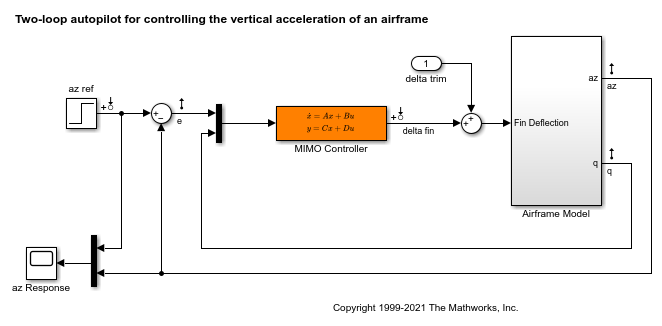evalGoal

Evaluate tuning goals for tuned control system

Description

example

[Hspec,fval] = evalGoal(Req,T) returns the normalized value fval of a tuning goal evaluated for a tuned control system T. The evalGoal command also returns the transfer function Hspec used to compute this value.

Examples

collapse all

Tune a control system with systune, and evaluate the tuning goals with evalGoal.

open_system('rct_airframe2')Create tracking, roll-off, stability margin, and disturbance rejection requirements for tuning the control system.

Req1 = TuningGoal.Tracking('az ref','az',1);
Req2 = TuningGoal.Gain('delta fin','delta fin',tf(25,[1 0]));
Req3 = TuningGoal.Margins('delta fin',7,45);
MaxGain = frd([2 200 200],[0.02 2 200]);
Req4 = TuningGoal.Gain('delta fin','az',MaxGain);

Create an slTuner interface, and tune the model using these tuning goals.

ST0 = slTuner('rct_airframe2','MIMO Controller');
rng default
[ST1,fSoft] = systune(ST0,[Req1,Req2,Req3,Req4]);
Final: Soft = 1.13, Hard = -Inf, Iterations = 94

ST1 is a tuned version of the slTuner interface to the control system. ST1 contains the tuned values of the tunable parameters of the MIMO controller in the model.

Evaluate the margin goal for the tuned system.

[hspec,fval] = evalGoal(Req3,ST1);
fval
fval =

0.5140

The normalized value of the tuning goal is less than 1, indicating that the tuned system satisfies the margin requirement. For more information about how the normalized value of this tuning goal is calculated, see the TuningGoal.Margins reference page.

Evaluate the tracking goal for the tuned system.

[hspec,fval] = evalGoal(Req1,ST1);
fval
fval =

1.1327

The tracking requirement is nearly met, but the value exceeds 1, indicating a small violation. To further assess the violation, you can use viewGoal to visualize the requirement against the corresponding response of the tuned system.

Input Arguments

collapse all

Tuning goal to evaluate, specified as a TuningGoal object or vector of TuningGoal objects. For a list of all TuningGoal objects, see Tuning Goals.

Tuned control system, specified as a generalized state-space (genss) model or an slTuner interface to a Simulink® model. T is typically the result of using the tuning goal to tune control system parameters with systune.

Example: [T,fSoft,gHard] = systune(T0,SoftReq,HardReq), where T0 is a tunable genss model

Example: [T,fSoft,gHard] = systune(ST0,SoftReq,HardReq), where ST0 is a slTuner interface object

Output Arguments

collapse all

Transfer function associated with the tuning goal, returned as a state-space (ss) model. evalGoal uses Hspec to compute the evaluated tuning goal, fval.

For example, suppose Req is a TuningGoal.Gain goal that limits the gain H(s) between some specified input and output to the gain profile w(s). In that case, Hspec is given by:

$Hspec\left(s\right)=\frac{1}{w\left(s\right)}H\left(s\right).$

fval is the peak gain of Hspec. If H(s) satisfies the tuning goal, fval <= 1.

For more information about the transfer function associated with the tuning goal, see the reference page for each tuning goal.

Normalized value of tuning requirement, returned as a positive scalar. The normalized value is a measure of how closely the requirement is met in the tuned system. The tuning requirement is satisfied if fval < 1. For information about how each type of TuningGoal requirement is converted into a normalized value, see the reference page for each tuning goal.

Tips

• For MIMO feedback loops, the LoopShape, MinLoopGain, MaxLoopGain, Margins, Sensitivity, and Rejection goals are sensitive to the relative scaling of each SISO loop. systune tries to balance the overall loop-transfer matrix while enforcing such goals. The optimal loop scaling is stored in the tuned closed-loop model or slTuner interface T returned by systune. For consistency, evalGoal(R,T) applies this same scaling when evaluating the tuning goals. To omit this scaling, use evalGoal(R,clearTuningInfo(T)).

Modifying T might compromise the validity of the stored scaling. Therefore, if you make significant modifications to T, retuning is recommended to update the scaling data.